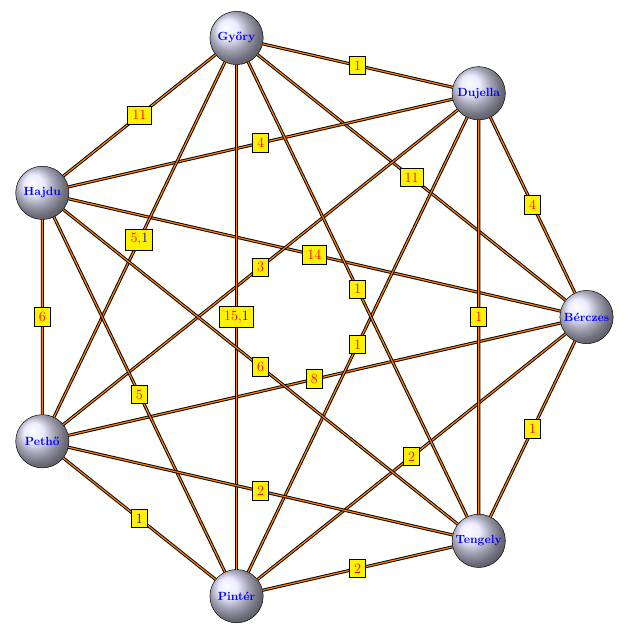## Collaboration $$K_7$$ graph

We define the collaboration graph $$G$$ of the research group as follows. The graph $$G$$ has as vertices all members of the research group and all co-authors of the members, with an edge joining $$u$$ and $$v$$ if $$u$$ and $$v$$ have published a joint paper. The maximal complete subgraph of $$G$$ is isomorphic to $$K_7$$.
Labels indicate the number of joint papers.
Blue numbers denote special article.